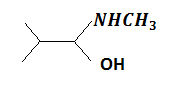Aldehydes and ketones will not form crystalline derivatives with

(a) sodium bisulphite

(b) phenyl hydrazine

(c) semicarbazide hydrochloride

(d) dihydrogen sodium phosphate

Complete Question Bank + Test Series
Complete Question Bank

Difficulty Level:

Acetone reacts with iodine $\left({\mathrm{I}}_{2}\right)$ to form iodoform in the presence of

(a) Ca${\mathrm{CO}}_{3}$          (b) NaOH          (c) KOH            (d) Mg${\mathrm{CO}}_{3}$

Complete Question Bank + Test Series
Complete Question Bank

Difficulty Level:

Which of the following compounds with molecular formula, C5H10 yields acetone on ozonolysis?

(a) 2-methyl-2-butene

(b) 3-methyl-1-butene

(c) Cyclopentane

(d) 2-methyl-1-butene

Complete Question Bank + Test Series
Complete Question Bank

Difficulty Level:

Among acetic acid, phenol and n-hexanol which one of the following compound will react with NaHCO3 solution to give sodium salt and CO2?

(a) Acetic acid

(b) n-hexanol

(c) Acetic acid and phenol

(d) Phenol

Complete Question Bank + Test Series
Complete Question Bank

Difficulty Level:

The reagent used for the separation of acetaldehyde from acetophenone is

(1) NaHSO3         (2) C6H5NHNH2            (3) NH2OH              (4) NaOH and I2

Complete Question Bank + Test Series
Complete Question Bank

Difficulty Level:

Iodoform test is not given by

(a) 2-pentanone                  (b) ethanol

(c) ethanal                          (d) 3-pentanone

Complete Question Bank + Test Series
Complete Question Bank

Difficulty Level:

Two isomeric ketones 3-Pentanones and 2-pentanone can be distinguished by

(a) I2/NaOH only               (b) NaHSO3 only

(c) NaCN/HCl                     (d) both of (a) and (b)

Complete Question Bank + Test Series
Complete Question Bank

Difficulty Level:

Products of the following reaction

CH3C$\equiv$C.CH2CH3  ... are

(a) CH3CHO + CH3CH2CHO

(b) CH3COOH + CH3COCH3

(c) CH3COOH + HOOC.CH2CH3

(d) CH3COOH + CO2

Complete Question Bank + Test Series
Complete Question Bank

Difficulty Level:

Which alkene on ozonolysis gives CH3CH2CHO and CH3COCH3?

(a) CH3CH2CH=C${<}_{{\mathrm{CH}}_{3}}^{{\mathrm{CH}}_{3}}$

(b) CH3CH2CH=CHCH2CH3

(c) CH3CH2CH=CHCH3

(d)

Complete Question Bank + Test Series
Complete Question Bank

Difficulty Level:

The major organic product formed from the following reaction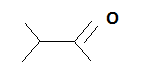... is

(a)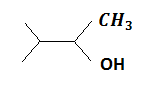(b)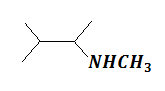(c)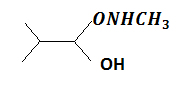(d)